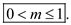# Quiz 3: Amplitude Modulation

The amplitude of the carrier signal varies according to the amplitude of the message signal is termed as amplitude modulation. The message signal is also called information signal. use figure 3.1 from the text book to observe the process of amplitude modulation. an AM signal can be generated by using the instantaneous amplitude of the base band signal to vary the carrier signal peak amplitude. The figure 3.1 shows amplitude modulation in part c which is obtained by combination of information signal with 1 kHz of sine wave shown in part a and the high frequency carrier signal with 10 kHz signal shown in part b. by joining the individual waveform peaks of the modulated signal, the resultant shape resembles that of original base band signal and this final shape is termed as envelope of the AM signal. this envelope repeats at the frequency of modulating signal. the shape of each positive or negative half is similar to that of base band signal. The AM signal envelope shows the information regarding the carrier signal. part c of text book figure 3.1 represents the 10 number of cycles of the carrier for each cycle of the base band signal. using the envelope of the AM signal one can determine the modulation index as shown below.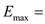maximum amplitude of the envelope of AM signal.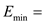minimum amplitude of the envelope of AM signal.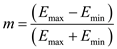Use figure 3.2 from the text book. It shows the envelope shape only, there is no possibility to see individual RF cycles. The ratio of carrier to base band signal is generally high as the frequency of the carrier is higher than base band signal. the figure 3.2 uses 1 MHz of carrier and 1 kHz of base band signal, the ratio of these two results a number 1000. It indicates the number of cycles of the carrier with respect to each cycle of the envelope. Hence, the AM signal envelope is significant for many applications.
In Amplitude Modulation (AM) the amplitude of the carrier signal varied in accordance with the amplitude of the message signal. The message signal is also known as the information signal or modulating signal or base band signal. The AM signal is generated by using the instantaneous amplitude of the base band signal to vary the carrier signal peak amplitude. If the peaks of the individual waveforms of the modulated signal are joined, the resulting envelope resembles the original modulating signal. Consider that the Amplitude modulated signal as shown in Figure 1 for the modulation index less than unity.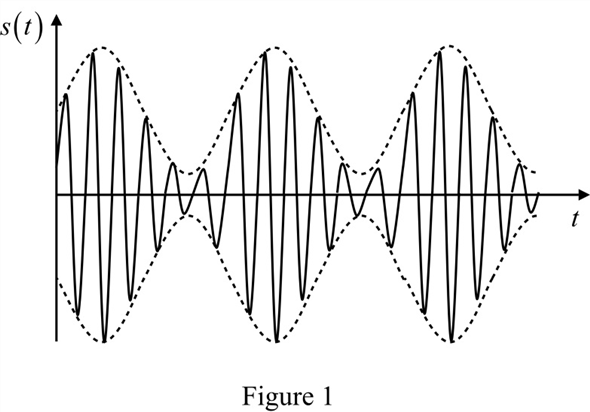Amplitude modulation is a nonlinear process. In this nonlinear interaction between the signals, sum and difference frequencies are generated and contain the information to be transmitted. Another interesting thing about AM is that we seem to be varying the amplitude of the carrier; in fact this is implied by the term Amplitude Modulation. But a look at frequency domain shows that the signal component at the carrier frequency survives intact, with the same amplitude and frequency as before. This is also shown in Figure 3.1 in the textbook. The Linear addition of two signals is shown in Figure 3.4 b in the textbook. At modulating frequency, no component of AM signal is observed. Total information is transmitted at frequencies that are nearer to the carrier. The diagram shows the information signal and carrier signal are remaining separate, at their original frequency. Therefore, the carrier signal is adjusted to get the shape of the envelope that resembles the information signal which is termed as modulation. Thus, although the amplitude modulation certainly involves changing the amplitude of the signal, it is not correct to say that the amplitude of the carrier is modulated.
Modulation index is defined as the ratio of amplitude of the base band signal to amplitude of the high frequency carrier signal. it is denoted by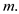by which amount the amplitude of a signal varied in modulation is measured by using the modulation index. It can be expressed in percentage also. for example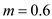means 60% of modulation. when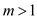then it indicates over modulation. use figure 3.6 from the text book to observe the effect of over modulation. for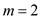the AM signal envelope does not resembles the original modulating signal. part a in figure 3.6 shows the waveform that is different from modulating signal. part c in figure 3.6 shows over modulation result for practical modulator. The waveform indicates zero output for negative modulation peaks. these peaks are necessary to indicate carrier components that are added to the base band signal. which is a draw back due to over modulation. The over modulation also generates the side band frequencies that are far from carrier frequency. this spurious frequencies are termed as splatter, which leads to the more bandwidth of modulated signal. and over modulation results distorted outputs. It gives a demodulated signal that is more in band width compared to original base band signal. which is another draw back. The value of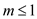results good envelope shape as well as the demodulated signal that is same as original information signal. for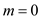the value of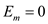and it results an un modulated carrier. When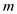changes between 0 and 1 the resultant waveforms can be observed in figure 3.5 from the text book. Hence to get good envelope shapeshould be as high as possible with out exceeding one. Hence, the modulation index of an AM signal should be as high as possible with out over modulation gives desirable outputs with out any distortion under the condition of